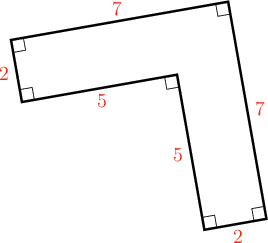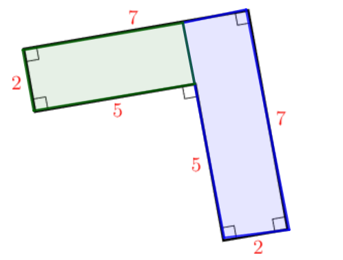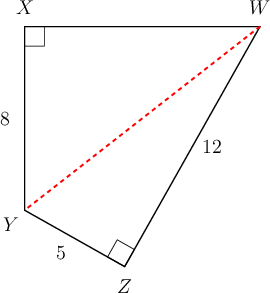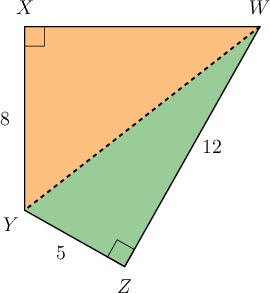Home Practice
For learners and parents For teachers and schools
Textbooks
Full catalogue
Pricing SupportLog in

We think you are located in United States. Is this correct?

# Area and perimeter of complex shapes

## Worked example 16.17: Calculating the area and perimeter of complex shapes

Determine the perimeter and area of the given shape. All lengths are measured in metres.### Determine the perimeter of the shape.

To find the perimeter of the shape, we need to find the sum of the lengths of the sides. Choose a starting point on the shape and then add the lengths of the sides:

Starting at the top right corner and moving in a clockwise direction:

$\text{Perimeter } = 7 + 2 + 5 + 5 + 2 + 7 = 28$

The perimeter of the shape is $$28 \text{ m}$$.

### Find the area of the shape.

To calculate the area, we divide the shape into two rectangles.\begin{align} \text{Area of shape } &= \text{area of green rectangle + area of blue rectangle} \\ &= (l \times b) + (l \times b) \\ &= (5 \times 2) + (7 \times 2) \\ &= 10 + 14 \\ &= 24 \\ \end{align}

The area of the shape is $$24 \text{ m}^2$$.

The perimeter of the shape is $$28 \text{ m}$$ and the area is $$24 \text{ m}^2$$.

## Worked example 16.18: Calculating the area of a polygon

In the diagram below, $$WZ = 12 \text{ m}, YZ = 5 \text{ m}, XY = 8 \text{ m}$$, and $$WY = 13 \text{ m}$$. Calculate the area and perimeter of quadrilateral $$WXYZ$$. Give your answer correct to one decimal place.### Divide the shape into two right-angled triangles.

We can divide the area of the quadrilateral into two right-angled triangles, $$\triangle WXY$$ with $$\angle X = 90°$$ and $$\triangle WYZ$$ with $$\angle Z = 90°$$.### Calculate the area of $$\triangle WYZ$$.

\begin{align} \text{Area of } \triangle WYZ &= \frac{1}{2}(b \times h) \\ &= \frac{1}{2}(5 \times 12) \\ &= \frac{1}{2}60 \\ &= 30 \\ \text{Area of } \triangle WYZ &= 30 \text{ m}^2 \end{align}

### Calculate the area of $$\triangle WXY$$.

For this triangle, we do not know the perpendicular height ($$XW$$), so we need to use the theorem of Pythagoras to calculate the length of $$XW$$:

\begin{align} WX^2 + XY^2 &= WY^2 \text{ (Pythagoras)} \\ WX^2 &= WY^2 − XY^2 \\ &= 13^2 − 8^2 \\ &= 169 − 64 \\ &= 105 \\ \therefore WX &= \sqrt{105} \end{align}

Now that we know the perpendicular height, we can calculate the area of $$\triangle WXY$$:

\begin{align} \text{Area of } \triangle WXY &= \frac{1}{2}(b \times h) \\ &= \frac{1}{2}(8 \times \sqrt{105}) \\ &= 4 \sqrt{105} \\ &= 4 \sqrt{105} = \text{40,9} \text{ m}^2 \end{align}

### Calculate the perimeter of $$WXYZ$$.

\begin{align} \text{Perimeter } WXYZ &= 12 + 5 + 8 + \sqrt{105} \\ &= \text{35,2} \\ &= \text{35,2} \text{ m} \end{align}

\begin{align} \text{Area of } WXYZ &= \text{area of } \triangle WYZ + \text{area of } \triangle WXY \\ &= 30 + \text{40,9} \\ &= \text{71,0} \text{ m}^2 \\ \text{Perimeter } WXYZ &= \text{35,2} \text{ m} \end{align}